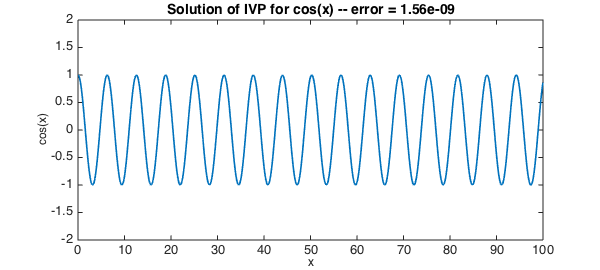This is an elementary example to illustrate how one might use Chebfun to solve an ODE initial-value problem. We take the world's second-simplest such problem,

$$u''+ u = 0 , ~~~~ u(0) = 1, ~ u'(0) = 0$$

on the interval $[0,100]$. The solution is $\cos(x)$.

d = [0,100];                  % domain
x = chebfun('x',d);           % x variable
L = chebop(d);                % name of operator
L.op = @(u) diff(u,2) + u;    % linear operator defining the ODE
L.lbc = @(u) [u-1;diff(u)];   % imposing Dirichlet and Neumann BCs
u = L\0;                      % solve the problem
plot(u,'linewidth',1.6)       % plot the solution
err = norm(u-cos(x),inf);     % measure the error
FS = 'fontsize';
xlabel('x',FS,12)
ylabel('cos(x)',FS,12)
title(sprintf('Solution of IVP for cos(x) -- error = %7.2e',err),FS,14)
ylim([-2 2])